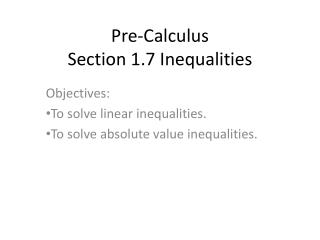# Pre-Calculus Section 1.7 Inequalities - PowerPoint PPT PresentationDownload PresentationPre-Calculus Section 1.7 Inequalities

Pre-Calculus Section 1.7 InequalitiesDownload Presentation## Pre-Calculus Section 1.7 Inequalities

- - - - - - - - - - - - - - - - - - - - - - - - - - - E N D - - - - - - - - - - - - - - - - - - - - - - - - - - -
##### Presentation Transcript

1. Pre-CalculusSection 1.7 Inequalities Objectives: To solve linear inequalities. To solve absolute value inequalities.

2. Solving an Inequality To solve an inequality that contains a variable means to find all values of that variable that make the inequality true. • Unlike an equation, an inequality generally has infinitely many solutions. • These form an interval or a union of intervals on the number line.

3. Ex 1. Solve the inequality. Express the solution using interval notation and graph the solution set. • 3x < 9x + 4 • 4 ≤ 3x – 2 < 13

4. Ex 2. Solve the absolute value inequality. Express the solution using interval notation and graph the solution set. a) b)

5. Class Work Solve, graph the solution set, and express the solution set in interval notation. • 5 – 3x ≤ -16 • -3 ≤ 3x + 7 ≤ ½ • 15x – 4 < 6

6. HW #6 p 84 7-27 eoo, 63-75 eoo, 95, 96